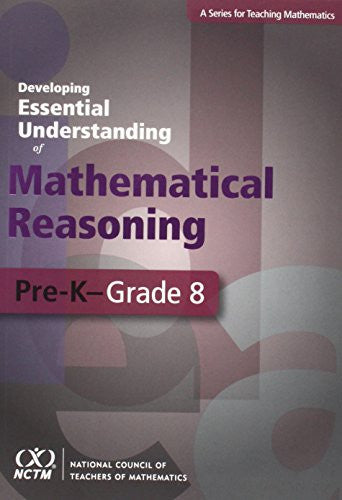Math Resources and GamesDeveloping Essential Understanding of Mathematical Reasoning for Teaching Mathematics in Grades Pre-K-8

\$32.95

Author: John Lannin

Edition: First

ISBN: 0873536665

Number Of Pages: 96

Publisher: National Council of Teachers of Mathematics

Details: How do your students determine whether a mathematical statement is true? Do they rely on a teacher, a textbook, or various examples? How can you encourage them to connect examples, extend their ideas to new situations that they have not yet considered, and reason more generally?

How much do you know...and how much do you need to know?

Helping your students develop a robust understanding of mathematical reasoning requires that you understand this mathematics deeply. But what does that mean?

This book focuses on essential knowledge for teachers about mathematical reasoning. It is organized around one big idea, supported by multiple smaller, interconnected ideas essential understandings.Taking you beyond a simple introduction to mathematical reasoning, the book will broaden and deepen your mathematical understanding of one of the most challenging topics for students and teachers. It will help you engage your students, anticipate their perplexities, avoid pitfalls, and dispel misconceptions. You will also learn to develop appropriate tasks, techniques, and tools for assessing students' understanding of the topic.

Focus on the ideas that you need to understand thoroughly to teach confidently.